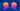Functions are basic constructs of programming language. This blog post we will show how to use them more effectivelyEffective Python is one the good books that is thought of to be Python 202. Once you’ve got the hang of basics, you’d like to look at ways to improve the code. This is exactly what this books provides.

Bret Saltkin gives a nice talk about this during PyCon 2015, titled How to Be More Effective with Functions. Following is summary of that talk

## Agenda

1. Reduce visual noise with variable positional arguments
2. Provide optional behaviour with keyword arguments
3. Enforce clarity with keyword-only argument
4. Consider generators instead of returning lists
5. Be defensive when iterating over arguments

## Reduce visual noise with variable positional arguments

This helps reduce visual clutter. Lets look at how the following snippet can be refactored

``````def log(message, values):
if not values:
print(message)
else:
out = ', '.join(str(x) for x in values)
print('%s: %s' % (message, out))

log("My numbers are", [1, 2])
log("Hi there", [])``````

Using positional argument we can do it as

``````def log(message, *values):
if not values:
print(message)
else:
out = ', '.join(str(x) for x in values)
print('%s: %s' % (message, out))

log("My numbers are", 1, 2)
log("Hi there")

# Alternative syntax to achieve same
# thing
nums = [1, 2, 3]
log("My numbers are", *nums)``````

This technique will not work with generators.

In summary:

1. Good for removing noise
3. Not for all APIs that accept sequence
4. Convenience for programmer

## Provide optional behaviour with keyword arguments

Lets say we have following function

``````def flow_rate(weight_diff, time_diff):
return weight_diff / time_diff``````

Now lets say we want to add extra functionality without affecting existing function calls we can do so by refactoring

``````def flow_rate(weight_diff, time_diff, period=3600, units_per_kg=2.2):
...``````

Note how `period` and `units_per_kg` are optional arguments that can be used for calculations

In summary:

1. Omit arguments you don’t need
2. New usage can access new functionality
3. No need to update existing callers

## Enforce clarity with keyword-only argument

In the functions definition above, you’re not really sure which one comes first, and which are optional {while making the call}. To solve this problem

``````def flow_rate(weight_diff, time_diff, *, period=3600, units_per_kg=2.2):
return (weight_diff * units_per_kg) / (time_diff * period)``````

Note the `*`, {BTW this is support for Python 3+}. What this implies is anything after `*` will be specified as keyword argument.

``flow = flow_rate(weight_diff, time_diff, period=60, units_per_kg=35.274)``

This is how the function will be getting executed, Optional arguments must use keywords

In summary:

1. Require clarity for optional arguments
2. Critically important for arguments of the same type {boolean flags, numbers}
3. Best way to extend *args functions

## Consider generators instead of returning lists

The default way to implement things should be generators as opposed to list. To understand lets take a look at following snippet:

``````def load_cities_list(path):
out = []
with open(path) as handle:
for line in handle:
city, count = line.split("\t")
out.append((city, int(count)))
return out``````

Some issues with the above snippet: You might run out of memory {where there is a huge file}. This can be re-written using following

``````def load_cities_list(path):
with open(path) as handle:
for line in handle:
city, count = line.split("\t")
yield city, int(count)``````

In summary:

1. Less noise, easier to read
2. File can be arbitrary size
3. Memory problems are gone

## Be defensive when iterating over arguments

``````def normalize(pop):
total = sum(x for _, x in pop)
for city, count in pop:
percent = 100 * count / total
yield city, percent``````

If you run the above code it will return `StopIteration` exception. This is because we’re iterating twice over pop.

This can be refactored into iterable container.

``````class LoadCities(object):

def __init__(self, path):
self.path = path

def __iter__(self):
with open(self.path) as handle:
for line in handle:
city, count = line.split()
yield city, int(count)``````

or

``````def normalize(pop):
total = sum(x for _, x in pop__iter__())
for city, count in pop.__iter__():
percent = 100 * count / total
yield city, percent``````

Note the extra `__iter__()`

In summary:

1. Beware of multiple iterations over the same argument
2. Define and use containers to avoid the problem entirely
3. Use `iter(x) is iter(x)` to detect non-container
4. Know the iterator protocol